# PSAT Math : How to find the diagonal of a prism

## Example Questions

### Example Question #1 : How To Find The Diagonal Of A Prism

The dimensions of a right, rectangular prism are 4 in x 12 in x 2 ft. What is the diagonal distance of the prism?

4√(3)

8√(7)

4√(46)

8√(23)

4√(46)

Explanation:

The problem is simple, but be carefulThe units are not equal. First convert the last dimension into inches. There are 12 inches per foot. Therefore, the prism's dimensions really are: 4 in x 12 in x 24 in.

From this point, things are relatively easy. The distance from corner to corner in a three-dimensional prism like this can be found by using a variation on the Pythagorean Theorem that merely adds one dimension. That is, d2  = x2y2 + z2, or d√(x2y2 + z2)

For our data, this would be:

d = √(42 + 122 + 242) = √(16 + 144 + 576) = √(736) = √(2 * 2 * 2 * 2 * 2 * 23) = 4√(46)

### Example Question #2 : How To Find The Diagonal Of A Prism

The base of a right, rectangular prism is a square. Its height is three times that of one of the sides of the base. If its overall volume is 375 in3, what is the diagonal distance of the prism?

25√(11) in

5 in

5√(11) in

5√(3) in

5√(11) in

Explanation:

First, let's represent our dimensions. We know the bottom could be represented as being x by x. The height is said to be three times one of these dimensions, so let's call it 3x. Based on this, we know the dimensions of the prism are x, x, and 3x. Now, the volume of a right rectangular prism is found by multiplying together its three dimensions. Therefore, if we know the overall volume is 375 in3, we can say:

375 = x * x * 3x or 375 = 3x3

Simplifying, we first divide by 3: 125 = x3. Taking the cube root of both sides, we find that x = 5.

Now, be careful. The dimensions are not 5, 5, 5. They are (recall) x, x, and 3x. If x = 5, this means the dimensions are 5, 5, and 15.

At this point, things are beginning to progress to the end of the problem. The distance from corner to corner in a three-dimensional prism like this can be found by using a variation on the Pythagorean Theorem that merely adds one dimension. That is, d2  = x2 + y2 + z2, or d = √(x2 + y2 + z2)

For our data, this would be: d = √(52 + 52 + 152) = √(25 + 25 + 225) = √(275) = √(5 * 5 * 11) = 5√(11) in

### Example Question #3 : How To Find The Diagonal Of A Prism

The base of a right, rectangular prism has one side that is three times the length of the other. Its height is twice the length of the longer side of the base. If its overall volume is 13,122 in3, what is the diagonal distance of the prism?

9√(23) in

9√(46) in

6√(23) in

9√(13) in

9√(46) in

Explanation:

First, let's represent our dimensions. We know the bottom could be represented as being x by 3x. The height is said to be twice the longer dimension, so let's call it 2 * 3x, or 6x. Based on this, we know the dimensions of the prism are x, 2x, and 6x. Now, the volume of a right rectangular prism is found by multiplying together its three dimensions. Therefore, if we know the overall volume is 13,122 in3, we can say:

13,122 = x * 3x * 6x or 13,122 = 18x3

Simplifying, we first divide by 18: 729 = x3. Taking the cube root of both sides, we find that x = 9.

Now, be careful. The dimensions are not 9, 9, and 9. They are (recall) x, 3x, and 6x. If x = 9, this means the dimensions are 9, 27, and 54.

At this point, things are beginning to progress to the end of the problem. The distance from corner to corner in a three-dimensional prism like this can be found by using a variation on the Pythagorean Theorem that merely adds one dimension. That is, d2  = x2 + y2 + z2, or d = √(x2 + y2 + z2)

For our data, this would be: d = √(92 + 272 + 542) = √(81 + 729 + 2916) = √(3726) = √(2 * 3 * 3 * 3 * 3 * 23) = 9√(46) in.

### Example Question #4 : How To Find The Diagonal Of A Prism

A rectangular prism has length 7, width 4, and height 4. What is the distance from the top back left corner to the bottom front right corner?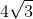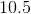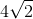Explanation:

The diagonal from the top back left corner to the bottom front right corner will be the hypotenuse of a right triangle. The sides of the triangle will be the height of the box and the diagonal through the middle of one of the rectangular faces. We will be able to solve for the length using the Pythagorean Theorem.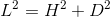To calculate the length of the hypotenuse, we first must find the length of the rectangular diagonal using the sides of the rectangle. This diagonal will be the hypotenuse of a right triangle with sides 7 and 4. Solve for the diagonal length using the Pythagorean Theorem.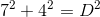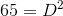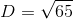Now we can return to our first triangle. We are given the height, 4, and now have the length of the rectangular diagonal. Use these values to solve for the length of the diagonal that connects the top back left corner and the bottom front right corner.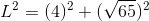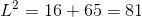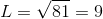### Example Question #1 : How To Find The Diagonal Of A Prism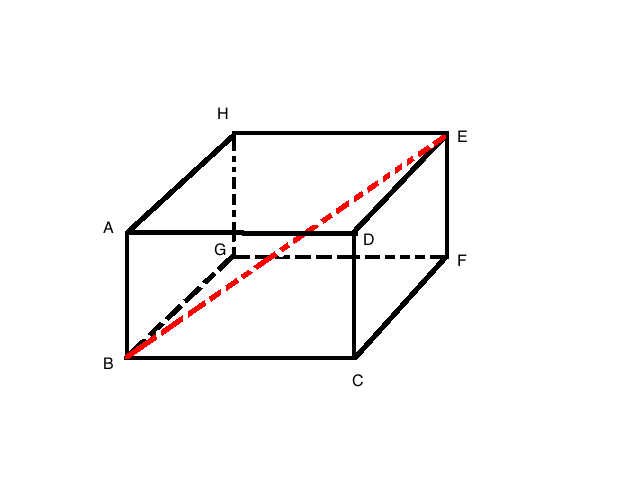In the rectangular prism above, the length of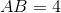,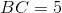, and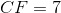. What is the length of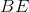?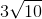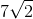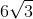Explanation:

The equation for the diagonal of a rectangular prism is

Diagonal=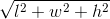When you plug in the values for the length, width, and height, you get

Diagonal=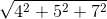Diagonal=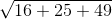Diagonal=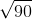Diagonal=